Anomalous Dynamics of Forced Translocation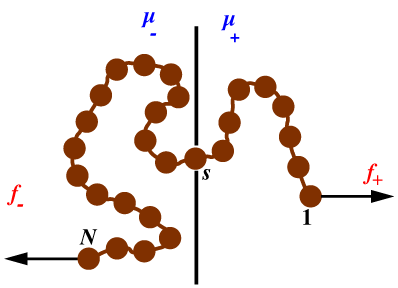Brownian dynamics:

• Polymer pulled by a force  f  exerted at one end: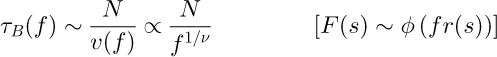• Polymer pulled by a chemical potential difference Δµ  between the two sides: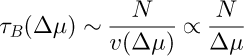• There is not enough time for equilibration at large N .

Lower bounds: How long does it take the forced polymer to translate by its typical size in the absence of the wall?

• Polymer pulled by a force  f  exerted at one end:
• Polymer pulled by a force Δµ  acting on a single monomer: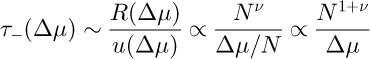Numerical results for the self-avoiding polymer in two dimensions:

• Polymer pulled by a force  f  exerted at one end: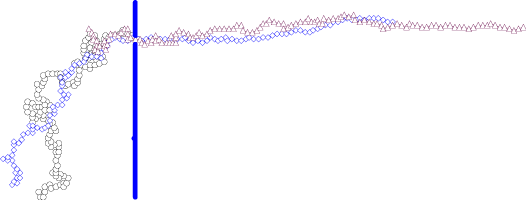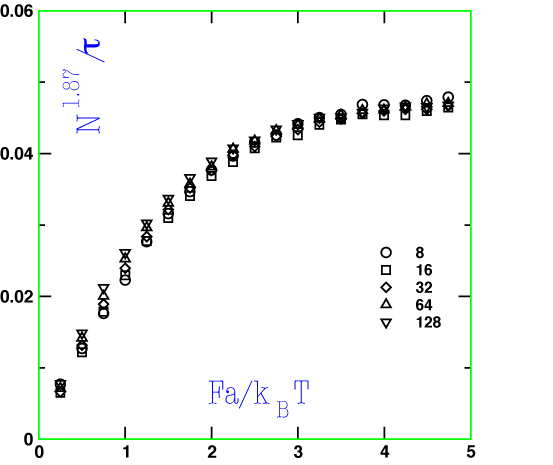• Polymer pulled by a chemical potential difference Δµ  between the two sides: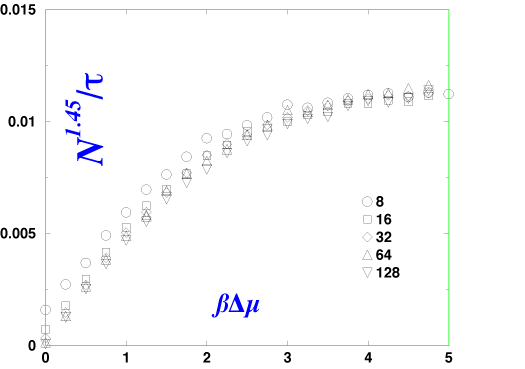• The numerically observed exponents appear to be lower than the lower bounds! (long crossovers?)